Home | | Strength of Materials II | Solved Problems: Civil - Strength of Materials - Energy Principles

# Solved Problems: Civil - Strength of Materials - Energy Principles

Civil - Strength of Materials - Energy Principles

Calculate the central deflection and the slope at ends of a simply supported beam carrying  a UDL w/ unit length over the whole span.

Solution:

a) Central deflection:

Since no point load is acting at the center where the deflection is required, apply the  fictitious load W, then the reaction at A andConsider a section at a distance x from A.

Bending moment at x,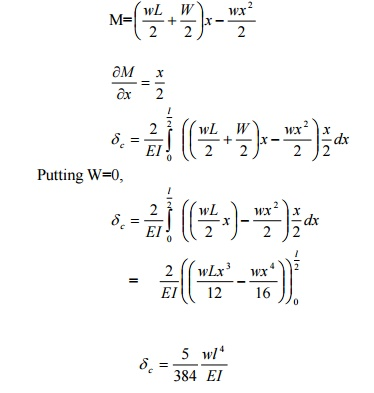b) Slope at ends

To obtain the slope at the end A, say apply a frictions moment A as shown in fig. The reactions at A and B will beWhere Mx is the moment at a point distant x from the origin (ie, B) is a function of M.A beam AB of span 3mis fixed at both the ends and carries a point load of 9 KN at C distant 1m  from A. The M.O.I. of the portion AC of the beam is 2I and that of portion CB is I. calculate the  fixed end moments and reactions.

Solution:

There are four unknowns Ma, Ra, Mb and Rb.Only two equations of static are available  (ie) v =0 and M =0

This problem is of second degree indeterminacy.

First choose MA and MB as redundant.1) For portion AC:

Taking A as the origin

Mx = -MA + RA xTo find MB, take moments at B, and apply the condition M =0 there. Taking clockwise  moment as positive and anticlockwise moment as negative. Taking MB clockwise, we have

MB -MA =RA (3) -9x2 = 0

MB -4.8 + (7.05x 3) -18 = 0

MB = 1.65 KN -m (assumed direction is correct)

To find RB Apply  V =0 for the whole frame.

RB = 9 -RA = 9-7.05 = 1.95 KN

Using Castigliano's First Theorem,rotationofthe determine  overhanging end A of the beam loaded.

Sol:

Rotation of A:

RB x L = -M

RB = -M/L

RB = M/L ( )

& RC = M/L ( )b) Deflection of A:

To find the deflection at A, apply a fictitious load W at A, in upward direction as

shown in fig.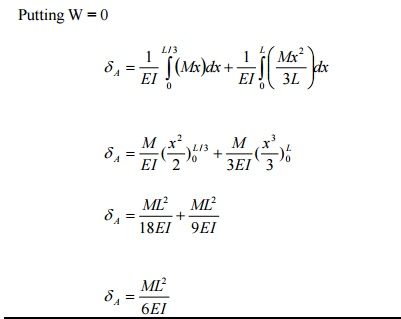Determine the vertical and horizontal displacements of the point C of the pin-jointed frame  shown in fig. The cross sectional area of AB is 100 sqmm and of AC and BC 150 mm2 each.  E= 2 x 10 5 N/mm2. (By unit load method)

Sol:

The vertical and horizontal deflections of the joint C are given byB) Stresses due to unit vertical load at C:

Apply unit vertical load at C. The Stresses in each member will be 1/6 than of those  obtained due to external load.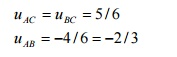C) Stresses due to unit horizontal load at C:

Assume the horizontal load towards left as shown in fig.The frame shown in fig. Consists of four panels each 25m wide, and the cross sectional  areas of the member are such that, when the frame carries equal loads at the panel points  of the lower chord, the stress in all the tension members is f n/mm2 and the stress in all the  comparison members of 0.8 f N/mm2.Determine the values of f if the ratio of the maximum  deflection to span is 1/900 Take E= 2.0 x 105 N/mm2.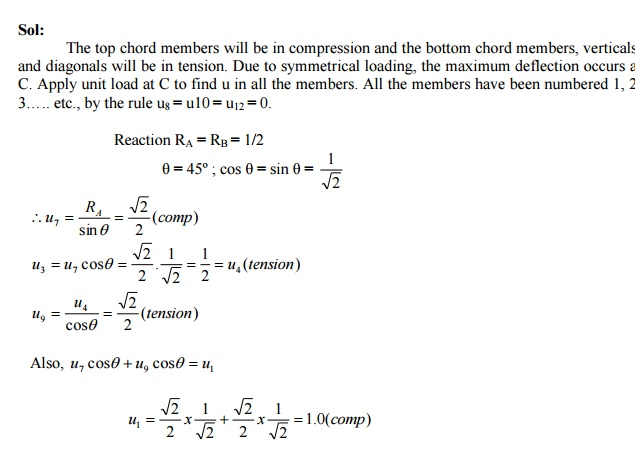Determine the vertical deflection of the joint C of the frame shown in fig. due to  temperature rise of 60� F in the upper chords only. The coefficient of expansion = 6.0 x 10-6  per 1� F and E = 2 x 10 6 kg /cm2.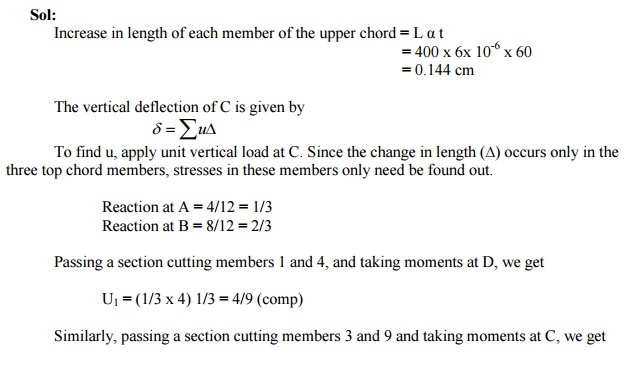Using the principle of least work, analyze the portal frame shown in Fig. Also plot the

B.M.D.

Sol:

The support is hinged. Since there are two equations at each supports. They are HA, VA, HD,  and VD. The available equilibrium equation is three. (i.e.) M =0, H =0, V =0 .

\ The structure is statically indeterminate to first degree. Let us treat the horizontal H ( ) at A  as redundant. The horizontal reaction at D will evidently be = (3-H) ( ). By taking moments at  D, we get

(VA x 3) + H (3-2) + (3 x 1) (2 -1.5) -(6 x 2) = 0

VA = 3.5 -H/3

VD = 6 -VA = 2.5 + H/3

By the theorem of minimum strain energy,(1)For member AB:

Taking A as the origin.(2) For the member BE:

Taking B as the origin.(3) For the member CE:

Taking C as the origin(4) For the member DC:

Taking D as the originA simply supported beam of span 6m is subjected to a concentrated load of 45 KN at 2m  from the left support. Calculate the deflection under the load point. Take E = 200 x 106  KN/m2 and I = 14 x 10-6 m4.RB = Total load -RA = 1/3 KN

Virtual Moment:

Consider section between AC

M1 = 2/3 X1   [limit 0 to 2]

Section between CB

M2 = 2/3 X2-1 (X2-2 ) [limit 2 to 6 ]

Real Moment:

M1= 30 x X1

M2 = 30 x X2 -45 (X2 -2)The deflection under the load = 57.1 mm

Define and prove the Maxwell's reciprocal theorem

The Maxwell?s reciprocal 'The work theorem done stated by the asf due to displacements caused by a second system of loads equals the work done by the second system of loads due to displacements caused by the first system o loads".

Maxwell?s theorem of reciprocal deflection  has the following three versions:

1. The deflection at A due to unit force at B is equal to deflection at B due to unit force at A.

?AB = ?BA

2. The slope at A due to unit couple at B is equal to the slope at B due to unit couple A

?AB = ?BA

3. The slope at A due to unit load at B is equal to deflection at B due to unit couple.Proof:Where,

M= bending moment at any point x due to external load.

m= bending moment at any point x due to unit load applied at the point where  deflection is required.

Let mXA=bending moment at any point x due to unit load at A

Let mXB = bending moment at any point x due to unit load at B.

M=mXA

To find deflection at B due to unit load at A, apply unit load at B.Then m= mXB

Hence,Similarly,

To find the deflection at A due to unit load at B, apply unit load at A.then m= mXAComparing (i) & (ii) we get

?AB = BA?

Using Castigliano's theorem, determine the de  shown in the fig. Take EI = 4.9 MN/m2.

Solution:

Apply dummy load W at B. Since we have to determine the deflection of the free end.  Consider a section xx at a distance x from B. Then

M x  =Wx +30(x-1)+20 *1* (x-1.5)+16(x-2)A cantilever, 8m long, carrying a point loads 5 KN at the center and an udl of 2 KN/m for a  length 4m from the end B. If EI is the flexural rigidity of the cantilever find the reaction at  the prop.' A simply supported beam of span L is carrying a concentrated load W at the centre and a uniformly  distributed load of intensity of w per unit leng  the centre of the beam.

Solution:

Let the load W is applied first and then the uniformly distributed load w.

Deflection due to load W at the centre of the beam is given byHence work done by W due to w is given by: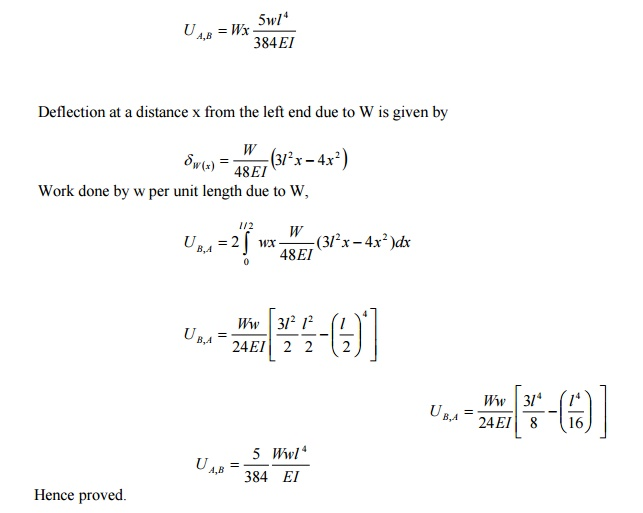Study Material, Lecturing Notes, Assignment, Reference, Wiki description explanation, brief detail
Civil : Strength of Materials : Energy Principles : Solved Problems: Civil - Strength of Materials - Energy Principles |